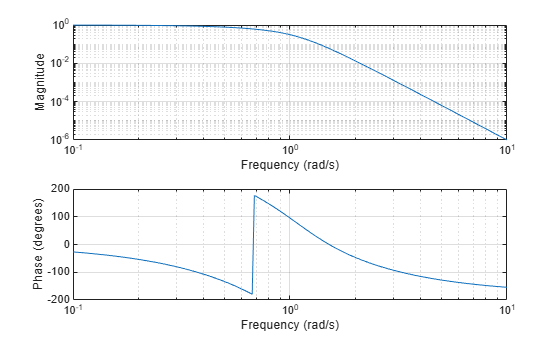# besselap

Bessel analog lowpass filter prototype

## Syntax

``[z,p,k] = besselap(n)``

## Description

example

````[z,p,k] = besselap(n)` returns the poles and gain of an order-`n` Bessel analog lowpass filter prototype.```

## Examples

collapse all

Design a 6th-order Bessel analog lowpass filter. Display its magnitude and phase responses.

```[z,p,k] = besselap(6); % Lowpass filter prototype [num,den] = zp2tf(z,p,k); % Convert to transfer function form freqs(num,den) % Frequency response of analog filter```## Input Arguments

collapse all

Filter order, specified as an integer scalar. `n` must be less than or equal to 25.

Data Types: `double`

## Output Arguments

collapse all

Zeros of the filter prototype, returned as an empty matrix. `z` is empty because there are no zeros.

Poles of the filter prototype, returned as a length-`n` column vector.

Gain of the filter prototype, returned as a scalar.

## Algorithms

The `besselap` function finds the filter roots from a lookup table constructed using Symbolic Math Toolbox™ software.

Analog Bessel filters are characterized by a group delay that is maximally flat at zero frequency and almost constant throughout the passband. The group delay at zero frequency is

`${\left(\frac{\left(2n\right)!}{{2}^{n}n!}\right)}^{1/n}.$`

The `besselap` function normalizes the poles and gain so that at low frequency and high frequency the Bessel prototype is asymptotically equivalent to the Butterworth prototype of the same order . The magnitude of the filter is less than $1/\sqrt{2}$ at the unity cutoff frequency Ωc = 1. The transfer function is expressed in terms of `z`, `p`, and `k` as

`$H\left(s\right)=\frac{k}{\left(s-p\left(1\right)\right)\left(s-p\left(2\right)\right)\cdots \left(s-p\left(n\right)\right)}.$`

 Rabiner, L. R., and B. Gold. Theory and Application of Digital Signal Processing. Englewood Cliffs, NJ: Prentice-Hall, 1975, pp. 228–230.